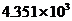Chapter 10, Problem 27QAP### Introductory Chemistry: A Foundati...

9th Edition
Steven S. Zumdahl + 1 other
ISBN: 9781337399425

#### Solutions

Chapter
Section### Introductory Chemistry: A Foundati...

9th Edition
Steven S. Zumdahl + 1 other
ISBN: 9781337399425
Textbook Problem
1 views

# Covert the following numbers of kilojoules into kilocalories. (Remember: kilo means 1000.) 652.1 kJ 1.00 kJ 4.184 kJ i>kJ

Interpretation Introduction

(a)

Interpretation:

Convert 652.1 kilojoules to kilocalories.

Concept Introduction:

A conversion factor is a numerical ratio which is used to show a measurement in one unit as another unit. It is always equal to 1.

Explanation

The given conversion is: conversion of kilojoules to kilocalories.

1 kilojoule is equal to 0.239006 kilocalorie.

1 kJ = 0.239006 kcal

Thus, conversion factor is given by:

1 kJ0.239006 kcal

So,

652

Interpretation Introduction

(b)

Interpretation:

Convert 1.00 kilojoules to kilocalories.

Concept Introduction:

A conversion factor is a numerical ratio which is used to show a measurement in one unit as another unit. It is always equal to 1.

Interpretation Introduction

(c)

Interpretation:

Convert 4.184 kilojoules to kilocalories.

Concept Introduction:

A conversion factor is a numerical ratio which is used to show a measurement in one unit as another unit. It is always equal to 1.

Interpretation Introduction

(d)

Interpretation:

Convert 4.351×103 kJ to kilocalories.

Concept Introduction:

A conversion factor is a numerical ratio which is used to show a measurement in one unit as another unit. It is always equal to 1.

### Still sussing out bartleby?

Check out a sample textbook solution.

See a sample solution

#### The Solution to Your Study Problems

Bartleby provides explanations to thousands of textbook problems written by our experts, many with advanced degrees!

Get Started